# PSEB 6th Class Maths Solutions Chapter 14 Data Handling Ex 14.1

Punjab State Board PSEB 6th Class Maths Book Solutions Chapter 14 Data Handling Ex 14.1 Textbook Exercise Questions and Answers.

## PSEB Solutions for Class 6 Maths Chapter 14 Data Handling Ex 14.1

1. The heights (in cm) of students of a class 6th were recorded as below:
116, 117, 125, 116, 118, 120, 125, 121, 124, 117, 116, 115, 119, 121, 124, 117, 116, 119, 123, 120, 116, 121, 119, 116, 118, 125, 116, 119, 123,122,121, 120
Arrange the data in a tabular form using tally marks.
Solution: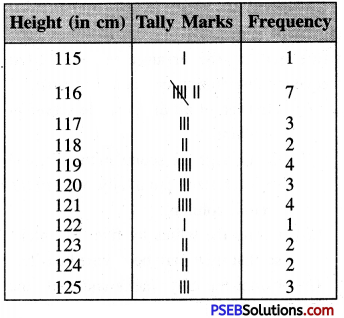2. The weight of 25 students (in kg) are given below:
25, 34, 32, 28, 25, 28, 34, 32, 32, 34, 32, 25, 28, 34, 34, 28, 28, 25, 32, 33, 32, 34, 33, 32,25
Arrange the data in a tabular form using tally marks.
Solution: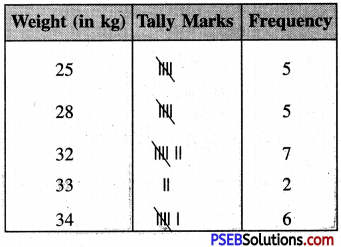3. Ekta is asked to collect data for size of shoes of students in her class 6th. Her finding are recorded in the manner shown below:Arrange the data in a tabular form using tally marks.
Solution: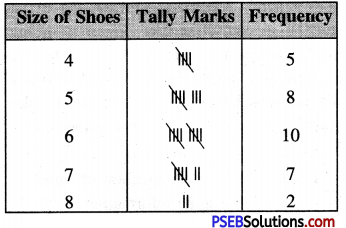4. Shweta threw a dice 40 times and noted the number appearing each time as shown below:Make a table and enter the data using tally marks. Find the number that appeared:

Question (i)
The minimum number of times.
Solution: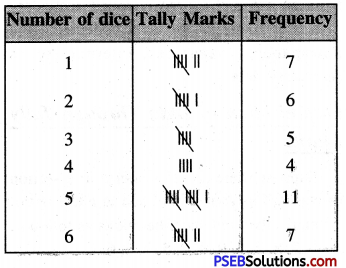4

Question (ii)
The maximum number of times.
Solution:
5.5. The students of class 6th had a Maths test and scored marks out of 10, which are listed below: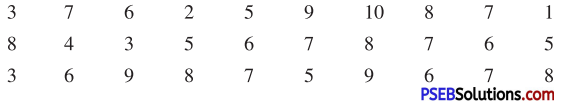Question (i)
Organize the data using tally marks.
Solution: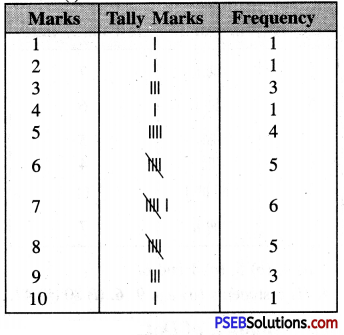Question (ii)
How many students scored less than or equal to 6?
Solution:
15Question (iii)
How many students scored more than 7?
Solution:
9